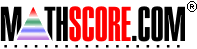Math Practice Online > free > lessons > Florida > 6th grade > Compare Rectangle Area and Perimeter

## Compare Rectangle Area and Perimeter

Calculate the rectangle area and perimeter. Express them in correct units.

 Sample Problems for Compare Rectangle Area and Perimeter Lesson for Compare Rectangle Area and Perimeter

### This topic aligns to the following state standards

Grade 4: Geo 2. Applies the concepts of area and perimeter to solve real-world and mathematical problems.
Grade 6: Meas 3. Solves real-world or mathematical problems involving perimeter or area and how these are affected by changes in the dimensions of the figure.

Copyright Accurate Learning Systems Corporation 2008.